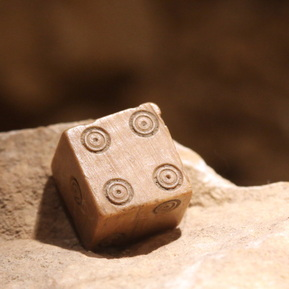# Winning At A Simple Game of Dice

Probability Level 2Calvin and David play a game of dice. They each throw 1 fair 6-sided dice. The probability that the number on David's dice is bigger than the number on Calvin's dice is $\frac {a}{b}$, where $a$ and $b$ are positive coprime integers. What is the value of $a+b$?

×